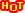# Ngoại ngữđoạn văn

#### mâypr0

##### Học sinh chăm học
Thành viên[TẶNG BẠN] TRỌN BỘ Bí kíp học tốt 08 mônChắc suất Đại học top - Giữ chỗ ngay!!

ĐĂNG BÀI NGAY để cùng trao đổi với các thành viên siêu nhiệt tình & dễ thương trên diễn đàn.

Students can make their reading ____ (1) effective by adopting a plan aimed at helping them to understand and to remember what they read. Firstly, they should decide precisely _____ (2) they're reading the book: perhaps it's to understand a difficult idea or argument. Then they should decide ____ (3) what they're going to read: a chapter of a book, for example.
It's helpful to get an overview of the contents before starting to read. This _____ (4) done by reading the introduction and the conclusion, and possibly skimming (or reading very quickly) some sections ____ (5) get a general idea of the contents.
1) A. much more
B. much less
C. less than
D. more than
2) A. why
B. where
C. what
D. how much
3) A. correctly
B. usually
C. exactly
D. rarely
4) A. must be
B. need to be
C. can be
D. will be
5) A. in order that
B. as
C. in order to
D. so that

#### Tzuyu-chan

##### Học sinh tiến bộ
HV CLB Địa lí
Thành viên
Students can make their reading ____ (1) effective by adopting a plan aimed at helping them to understand and to remember what they read. Firstly, they should decide precisely _____ (2) they're reading the book: perhaps it's to understand a difficult idea or argument. Then they should decide ____ (3) what they're going to read: a chapter of a book, for example.
It's helpful to get an overview of the contents before starting to read. This _____ (4) done by reading the introduction and the conclusion, and possibly skimming (or reading very quickly) some sections ____ (5) get a general idea of the contents.
1) A. much more
B. much less
C. less than
D. more than
2) A. why
B. where
C. what
D. how much
3) A. correctly
B. usually
C. exactly
D. rarely
4) A. must be
B. need to be
C. can be
D. will be
5) A. in order that
B. as
C. in order to
D. so that
1) A. much more
B. much less
C. less than
D. more than
2) A. why
B. where
C. what
D. how much
3) A. correctly
B. usually
C. exactly
D. rarely
4) A. must be
B. need to be
C. can be
D. will be
5) A. in order that
B. as
C. in order to
D. so that
bạn tham khảo nha chúc bạn học tốt !!

•phuongdaitt1